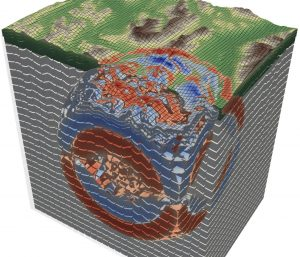# Simulation of Fluids and Wave Phenomena - High Order, High Performance, High Productivity

Dozenten:
C.-D. Munz, Stuttgart

Gastdozent:
D. Frey, Erlangen
H. Köstler, Erlangen

The simulation of such diverse problems as the propagation of seismic waves or tsunamis, the flows of compressible fluids (in atmosphere or around planes) or even electro-magnetic effects are all based on very similar mathematical models: hyperbolic systems of partial differential equations.

Interestingly, such hyperbolic systems can be solved via pretty similar numerical approaches. We will discuss the basics of these techniques, from Finite Volume schemes to discontinuous Galerkin methods, in which solutions are expressed via high-order polynomial in each cell of a computational mesh.

In the second half of the course, we will strive to implement several different scenarios modeled by such hyperbolic systems, using a generic engine to solve hyperbolic PDEs.